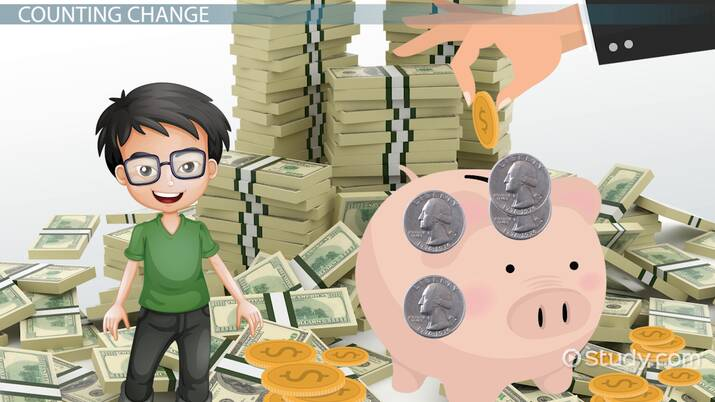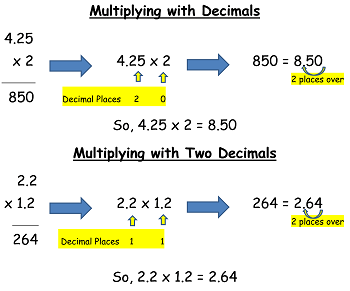# Multiplying Decimals: Lesson for Kids

Lesson Transcript
Instructor: Rayna Cummings

Rayna has taught Elementary Education for 12 years (in both 1st, 2nd, and 3rd grades) and holds a M.Ed in Early Childhood Education from The Ohio State University

While multiplying by decimals may sound difficult, it's actually no different than multiplying whole numbers. In this lesson, you'll learn about the extra step used when multiplying decimals by 10, 100 and even 1000! Updated: 05/26/2020

## Counting Change

Money is great, right? If you have enough in your piggy bank, you can buy that awesome toy you've been saving up for. But did you know that money is also great for teaching how to work with decimals? For example, you just broke open that piggy bank and found four quarters. A quarter equals \$0.25 cents, so four quarters would equal 4 x \$0.25 or \$1.00? You just multiplied decimals!An error occurred trying to load this video.

Try refreshing the page, or contact customer support.

Coming up next: Dividing Decimals: Steps, Rules & Examples

### You're on a roll. Keep up the good work!

Replay
Your next lesson will play in 10 seconds
• 0:04 Counting Change
• 0:30 Multiplying Decimals
• 1:26 Multiplying by 10, 100, & 1000
• 2:56 Lesson Summary
Save Save

Want to watch this again later?

Timeline
Autoplay
Autoplay
Speed Speed

## Multiplying Decimals

While multiplying decimals may sound tricky, it really isn't. You can multiply decimals just the way you multiply whole numbers. When you have your product, or the result of multiplying two numbers, you'll have to deal with the decimal. Let's take a look at the steps for multiplying and dealing with decimals.

Step 1: Multiply the numbers as if the decimal point isn't there.

• You can determine where to place the decimal point by counting the total number of decimal places in the factors, or numbers being multiplied. Count the number of decimal places by moving backwards, from right to left, from the end of each factor.

Let's take a look at a couple of examples:Did you notice how we ignored the decimal point when multiplying? Then we counted the total number of decimal places in the factors and placed the decimal point in the answer, moving backwards from the end of the answer.

## Multiplying by 10, 100, & 1,000

You may already know that when multiplying a number by 10, 100, or even 1,000, the easiest way to do that is to count the number of zeros and add that figure to the original number. For example, 6 x 100 = 600. Here, we just added the two zeros from the 100 to the 6.

To unlock this lesson you must be a Study.com Member.

### Register to view this lesson

Are you a student or a teacher?